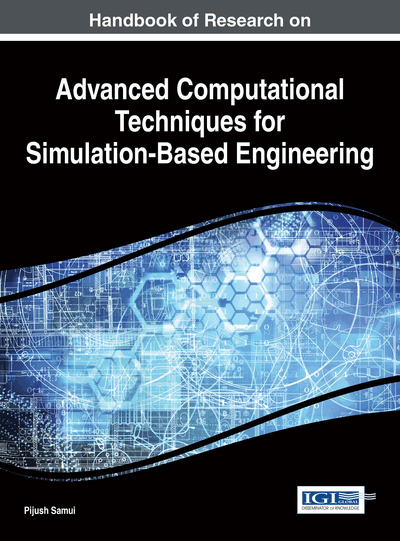# Variable Significance in the Determination of Friction Factor in Alluvial Channel

Kumar B (Indian Institute of Technology, India) and Sreenivasulu G (RGM College of Engineering and Technology, India)
DOI: 10.4018/978-1-4666-9479-8.ch018

## Abstract

The problem of predicting flow resistance in alluvial channels with sufficient accuracy is of great interest to hydraulic engineers. As the process is extremely complex, getting deterministic or analytical form of process phenomena is too difficult. Neural network modeling (ANN), which is particularly useful in modeling processes about which adequate knowledge of the physics is limited, is presented here as a tool complimentary to predict the complex non-linear relationship between the friction factor of an alluvial channel and its influencing factors. Friction factor comprises of different hydraulic and geometric parameters. Hence it is important to know the influences of these parameters on friction factor. Based on the input significant techniques through ANN model, it has been found that flow velocity influences more friction factor. The least influencing parameter is channel width. This shows the importance of determining the flow velocity more correctly.
Chapter Preview
Top

## 2. Theoretical Background

The friction factor in a straight channel with flowing sediment is a function of the flow characteristics, the geometry of the channel cross section and the properties of the fluid and sediment. For steady flow the Darcy-Weisbach coefficient λ can be expressed as (Yen, 2002, Kumar and Rao 2010):

(1) where ρ and μ are density and dynamic viscosity of the fluid; ρs and d are density and mean size of the sediment particles respectively; g is the gravitational acceleration; sf is the energy slope; u and y are mean velocity and flow depth of the flow and ζ represents a cross-sectional shape factor. The cross-sectional shape factor can be represented by b/y, where b is the width of the channel (Yen, 2002). Thus the functional relationship of friction factor can be approximated as:

## Complete Chapter List

Search this Book:
Reset